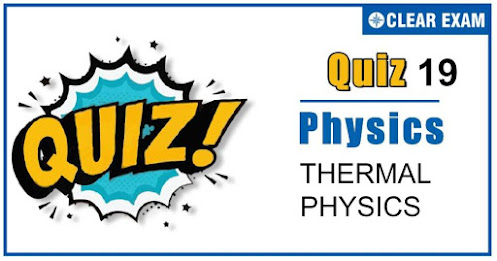## [LATEST]\$type=sticky\$show=home\$rm=0\$va=0\$count=4\$va=0

As per analysis for previous years, it has been observed that students preparing for NEET find Physics out of all the sections to be complex to handle and the majority of them are not able to comprehend the reason behind it. This problem arises especially because these aspirants appearing for the examination are more inclined to have a keen interest in Biology due to their medical background. Furthermore, sections such as Physics are dominantly based on theories, laws, numerical in comparison to a section of Biology which is more of fact-based, life sciences, and includes substantial explanations. By using the table given below, you easily and directly access to the topics and respective links of MCQs. Moreover, to make learning smooth and efficient, all the questions come with their supportive solutions to make utilization of time even more productive. Students will be covered for all their studies as the topics are available from basics to even the most advanced. .

Q1. An aluminium sphere of 20 cm diameter is heated from 0°C to 100°C.Its volume changes by (given that coefficient of linear expansion for aluminium(αAl=23×10-6/℃) :
•  28.9 cc
•  2.89 cc
•  9.28 cc
•  49.8 cc
Solution
Cubical expansion ∆V = γV∆T ∆V = 3αV∆T = 3×23×10-6× (4/3 π×103 )×100 = 28.9cc

Q2. Two spherical black bodies of radii r1 and r2 and with surface temperature T1 and T2 respectively radiate the same power. Then the ratio of r1 and r2 will be
•  (T2/T1 )2
•  (T2/T1 )4
•  (T1/T2 )2
•  (T1/T2 )4
Solution
For black body, P=AεσT4. For same power A∝1/T4 ⇒(r1/r2 )2=(T2/T1 )4⇒r1/r2 =(T2/T1 )4

Q3. The ratio of energy of emitted radiation of a black body at 27℃ and 927℃ is
•  1 : 4
•  1 : 16
•  1 : 64
•  1 : 256
Solution
Q1/Q2 =(T1/T2 )4=((273+27)/(273+927))4=(1/4)4=1/256

Q4. If the temperature difference on the two sides of a wall increases from 100℃ to 200℃, its thermal conductivity?
•  Remains unchanged
•  Is doubled
•  Is halved
•  Becomes four times
Solution
Thermal conductivity is independent of temperatures of the wall, it is a constant for the material, so it will remain unchanged

Q5. When the temperature of a rod increases from t to t+∆t, its moment of inertia increases from I to I+∆I. If α be the coefficient of linear expansion of the rod, then the value of ∆I/I is.
•  2α∆t
•  α∆t
•  α∆t/2
•  ∆t/α
Solution
Moment of inertia of a rod, I=1/12 ML2…(i) Where M is the mass of the rod and L is the length of the rod ∴∆I=1/12 2ML∆L (∵M is a constant)…(ii) Divide (ii) by (i), we get ∆I/I=2∆L/L…(iii) As ∆L=L α∆t Or ∆L/L=α∆t Substituting the value of ∆L/L in (iii), we get ∆I/I=2α∆t

Q6. A solid sphere and a hollow sphere of the same material and size are heated to the same temperature and allowed to cool in the same surroundings. If the temperature difference between each sphere and its surroundings is T, then?
•  The hollow sphere will cool at a faster rate for all values of T(
•  The solid sphere will cool at a faster rate for all values of T
•  Both spheres will cool at the same rate for all values of T
•  Both spheres will cool at the same rate only for small values of T
Solution
Rate of cooling ∆θ/t=(Aεσ(T4-T04))/mc As surface area, material and temperature difference are same, so rate of loss of heat is same in both, the spheres. Now in this case rate of cooling depends on mass ⇒ Rate of cooling ∆θ/t∝1/m ∵msolid>mhollow. Hence hollow sphere will cool fast .

Q7. Two circular discs A and B with equal radii are blackened. They are heated to some temperature and are cooled under identical conditions. What inference do you draw from their cooling curves?
•  A and B have same specific heats
•  Specific heat of A is less
•  Specific heat of B is less
•  Nothing can be said
Solution
According to Newton’s law of cooling, rate of cooling is given by ((-dT)/dt)=eAσ/mc(T4-T04) wherec is specific heat of material. or ((-dT)/dt)∝1/c ie., rate of cooling varies inversely as specific heat. From the graph, for A rate of cooling is larger. Therefore, specific heat of A is smaller.

Q8. A quantity of heat required to change the unit mass of a solid substance, from solid state to liquid state, while the temperature remains constant, is known as
•  Latent heat
•  Sublimation
•  Hoar frost
•  Latent heat of fusion
Solution
Latent heat of fusion

Q9. A black body emits radiations of maximum intensity for the wavelength of 5000Å when the temperature of the body is 1227℃. If the temperature of the body is increased by 1000℃, the maximum intensity would be observed at
•  1000Å
•  2000Å
•  5000Å
•  3000Å
Solution
According to Wien’s displacement law, λm T=constant or λm∝1/T or (λm )1/(λm )2 =T2/T1 ∴5000/(λm )2 =(2227+273)/(1227+273) or 5000/(λm )2 =2500/1500 ∴(λm )2=3000 Å

Q10. The maximum wavelength of radiation emitted at 2000K is 4μm. What will be the maximum wavelength of radiation emitted at 2400 K?:
•  3.33μm
•  0.66μm
•  1μm
•  1m
Solution
∴λ(m2 )(m1 ) =T1/T2 ⇒λ(m2 )=2000/2400×4=3.33 μm## Want to know more

Please fill in the details below:

## Latest NEET Articles\$type=three\$c=3\$author=hide\$comment=hide\$rm=hide\$date=hide\$snippet=hide

Name

ltr
item
BEST NEET COACHING CENTER | BEST IIT JEE COACHING INSTITUTE | BEST NEET & IIT JEE COACHING: Thermal Physics Quiz 19
Thermal Physics Quiz 19
https://1.bp.blogspot.com/-5a-vUR0zaYk/X5cQMXcavfI/AAAAAAAABuE/1oswBrXghJYEV4ceEPCwyKkA6uDzTgPUwCLcBGAsYHQ/w497-h261/Quiz%2BImage%2BTemplate%2B%252818%2529%2B%25281%2529.jpg
https://1.bp.blogspot.com/-5a-vUR0zaYk/X5cQMXcavfI/AAAAAAAABuE/1oswBrXghJYEV4ceEPCwyKkA6uDzTgPUwCLcBGAsYHQ/s72-w497-c-h261/Quiz%2BImage%2BTemplate%2B%252818%2529%2B%25281%2529.jpg
BEST NEET COACHING CENTER | BEST IIT JEE COACHING INSTITUTE | BEST NEET & IIT JEE COACHING
https://www.cleariitmedical.com/2020/10/ThermalPhysicsQuiz19.html
https://www.cleariitmedical.com/
https://www.cleariitmedical.com/
https://www.cleariitmedical.com/2020/10/ThermalPhysicsQuiz19.html
true
7783647550433378923
UTF-8

STAY CONNECTED